## Agile vs. Lean: Explained by CatsOver the past few years, Agile has gained popularity. This methodology emerged as a solution to manage projects with a number of unknown elements and to counter the typical waterfall method. Quality practitioners have observed the numerous similarities between this new framework and Lean. Some have speculated that Agile is simply the next generation’s version of Lean. These observations have posed the question: Is Agile the new Lean?

ASQ Influential Voices Roundtable for December 2019

The short answer to this question is: NO.

The longer answer is one I’m going to have to hold back some emotions to answer. Why? I have two reasons.

## Reason #1: There is No Magic Bullet

First, many managers are on a quest for the silver bullet — a methodology or a tool that they can implement on Monday, and reap benefits no later than Friday. Neither lean nor agile can make this happen. But it’s not uncommon to see organizations try this approach. A workgroup will set up a Kanban board or start doing daily stand-up meetings, and then talk about how they’re “doing agile.” Now that agile is in place, these teams have no reason to go any further.

## Reason #2: There is Nothing New Under the Sun

Neither approach is “new” and neither is going away. Lean principles have been around since Toyota pioneered its production system in the 1960s and 1970s. The methods prioritized value and flow, with attention to reducing all types of waste everywhere in the organization. Agile emerged in the 1990s for software development, as a response to waterfall methods that couldn’t respond effectively to changes in customer requirements.

Agile modeling uses some lean principles: for example, why spend hours documenting flow charts in Visio, when you can just write one on a whiteboard, take a photo, and paste it into your documentation? Agile doesn’t have to be perfectly lean, though. It’s acceptable to introduce elements that might seem like waste into processes, as long as you maintain your ability to quickly respond to new information and changes required by customers. (For example, maybe you need to touch base with your customers several times a week. This extra time and effort is OK in agile if it helps you achieve your customer-facing goals.)

Both lean and agile are practices. They require discipline, time, and monitoring. Teams must continually hone their practice, and learn about each other as they learn together. There are no magic bullets.

Information plays a key role. Effective flow of information from strategy to action is important for lean because confusion (or incomplete communication) and forms of waste. Agile also emphasizes high-value information flows, but for slightly different purposes — that include promoting:

• Rapid understanding
• Rapid response
• Rapid, targeted, and effective action

The difference is easier to understand if you watch a couple cat videos.

## This Cat is A G I L E

This cat is continuously scanning for information about its environment. It’s young and in shape, and it navigates its environment like a pro, whizzing from floor to ceiling. If it’s about to fall off something? No problem! This cat is A G I L E and can quickly adjust. It can easily achieve its goal of scaling any of the cat towers in this video. Agile is also about trying new things to quickly assess whether they will work. You’ll see this cat attempt to climb the wall with an open mind, and upon learning the ineffectiveness of the approach, abandoning that experiment.

## This Cat is L E A N

This cat is using as LITTLE energy as possible to achieve its goal of hydration. Although this cat might be considered lazy, it is actually very intelligent, dynamically figuring out how to remove non-value-adding activity from its process at every moment. This cat is working smarter, not harder. This cat is L E A N.

Hope this has been helpful. Business posts definitely need more cat videos.

## An Easy Way to Make Minimum Viable Product (MVP) Totally Not ViableThe Minimum Viable Product (MVP) concept has taken off over the past few years. Indeed, its heart is in the right place. MVP encourages product managers to scope features and functionality carefully so that customer needs are satisfied at every stage of development — not just in a sweeping finale at the end of development.

It’s a great way to shorten time-to-value and test new market concepts before committing. Zappos, for example, started by posting pictures of shoes on the internet without having an inventory. They wanted to quickly test to see whether people would even consider buying shoes without trying them on.

Unfortunately, adhering to MVP won’t guarantee success thanks to one critical caveat. And that is: if your product already exists, you have to consider your product’s base state. What can your customers do right now with your product? Failure to take this into consideration can be disastrous.

### An Example: Your Web Site

Here’s what I mean: let’s say the product is your company’s web site. If you’re starting from scratch, a perfectly suitable MVP would be a splash page with one or two sentences about what you do. Maybe you’d add some contact information. Customers will be able to find you and communicate with you, and you’ll be providing greater value than without a web presence.

But if you already have a 5000-page site online, that solution is not going to fly. Customers and prospects returning to your site will wonder why it vaporized. If they’re relying on the content or functionality you previously provided, chances are they will not be happy. Confused, they may choose to go elsewhere.

The moral of the story is: in defining the scope of your MVP, take into consideration what your customers can already do, and don’t dare give them less in your next release.

## Imperfect Action is Better Than Perfect Inaction: What Harry Truman Can Teach Us About Loss Functions (with an intro to ggplot)One of the heuristics we use at Intelex to guide decision making is former US President Truman’s advice that “imperfect action is better than perfect inaction.” What it means is — don’t wait too long to take action, because you don’t want to miss opportunities. Good advice, right?

When I share this with colleagues, I often hear a response like: “that’s dangerous!” To which my answer is “well sure, sometimes, but it can be really valuable depending on how you apply it!” The trick is: knowing how and when.

Here’s how it can be dangerous. For example, statistical process control (SPC) exists to keep us from tampering with processes — from taking imperfect action based on random variation, which will not only get us nowhere, but can exacerbate the problem we were trying to solve. The secret is to apply Truman’s heuristic based on an understanding of exactly how imperfect is OK with your organization, based on your risk appetite. And this is where loss functions can help.

Along the way, we’ll demonstrate how to do a few important things related to plotting with the ggplot package in R, gradually adding in new elements to the plot so you can see how it’s layered, including:

• Plot a function based on its equation
• Add text annotations to specific locations on a ggplot
• Draw horizontal and vertical lines on a ggplot
• Draw arrows on a ggplot
• Add extra dots to a ggplot
• Eliminate axis text and axis tick marks

## What is a Loss Function?

A loss function quantifies how unhappy you’ll be based on the accuracy or effectiveness of a prediction or decision. In the simplest case, you control one variable (x) which leads to some cost or loss (y). For the case we’ll examine in this post, the variables are:

• How much time and effort you put in to scoping and characterizing the problem (x); we assume that time+effort invested leads to real understanding
• How much it will cost you (y); can be expressed in terms of direct costs (e.g. capex + opex) as well as opportunity costs or intangible costs (e.g. damage to reputation)

Here is an example of what this might look like, if you have a situation where overestimating (putting in too much x) OR underestimating (putting in too little x) are both equally bad. In this case, x=10 is the best (least costly) decision or prediction:

``````# describe the equation we want to plot
parabola <- function(x) ((x-10)^2)+10

# initialize ggplot with a dummy dataset
library(ggplot)
p <- ggplot(data = data.frame(x=0), mapping = aes(x=x))

p + stat_function(fun=parabola) + xlim(-2,23) + ylim(-2,100) +
xlab("x = the variable you can control") +
ylab("y = cost of loss (\$\$)")``````

In regression (and other techniques where you’re trying to build a model to predict a quantitative dependent variable), mean square error is a squared loss function that helps you quantify error. It captures two facts: the farther away you are from the correct answer the worse the error is — and both overestimating and underestimating is bad (which is why you square the values). Across this and related techniques, the loss function captures these characteristics:

Not all loss functions have that general shape. For classification, for example, the 0-1 loss function tells the story that if you get a classification wrong (x < 0) you incur all the penalty or loss (y=1), whereas if you get it right (x > 0) there is no penalty or loss (y=0):

``````# set up data frame of red points
d.step <- data.frame(x=c(-3,0,0,3), y=c(1,1,0,0))

# note that the loss function really extends to x=-Inf and x=+Inf
ggplot(d.step) + geom_step(mapping=aes(x=x, y=y), direction="hv") +
geom_point(mapping=aes(x=x, y=y), color="red") +
xlab("y* f(x)") + ylab("Loss (Cost)") +
ggtitle("0-1 Loss Function for Classification")``````

## Use the Loss Function to Make Strategic Decisions

So let’s get back to Truman’s advice. Ideally, we want to choose the x (the amount of time and effort to invest into project planning) that results in the lowest possible cost or loss. That’s the green point at the nadir of the parabola:

``````p + stat_function(fun=parabola) + xlim(-2,23) + ylim(-2,100) +
xlab("Time Spent and Information Gained (e.g. person-weeks)") + ylab("\$\$ COST \$\$") +
annotate(geom="text", x=10, y=5, label="Some Effort, Lowest Cost!!", color="darkgreen") +
geom_point(aes(x=10, y=10), colour="darkgreen")``````

Costs get higher as we move up the x-axis:

``````p + stat_function(fun=parabola) + xlim(-2,23) + ylim(-2,100) +
xlab("Time Spent and Information Gained (e.g. person-weeks)") + ylab("\$\$ COST \$\$") +
annotate(geom="text", x=10, y=5, label="Some Effort, Lowest Cost!!", color="darkgreen") +
geom_point(aes(x=10, y=10), colour="darkgreen") +
annotate(geom="text", x=0, y=100, label="\$\$\$\$\$", color="green") +
annotate(geom="text", x=0, y=75, label="\$\$\$\$", color="green") +
annotate(geom="text", x=0, y=50, label="\$\$\$", color="green") +
annotate(geom="text", x=0, y=25, label="\$\$", color="green") +
annotate(geom="text", x=0, y=0, label="\$ 0", color="green")``````

And time+effort grows as we move along the x-axis (we might spend minutes on a problem at the left of the plot, or weeks to years by the time we get to the right hand side):

``````p + stat_function(fun=parabola) + xlim(-2,23) + ylim(-2,100) +
xlab("Time Spent and Information Gained (e.g. person-weeks)") + ylab("\$\$ COST \$\$") +
annotate(geom="text", x=10, y=5, label="Some Effort, Lowest Cost!!", color="darkgreen") +
geom_point(aes(x=10, y=10), colour="darkgreen") +
annotate(geom="text", x=0, y=100, label="\$\$\$\$\$", color="green") +
annotate(geom="text", x=0, y=75, label="\$\$\$\$", color="green") +
annotate(geom="text", x=0, y=50, label="\$\$\$", color="green") +
annotate(geom="text", x=0, y=25, label="\$\$", color="green") +
annotate(geom="text", x=0, y=0, label="\$ 0", color="green") +
annotate(geom="text", x=2, y=0, label="minutes\nof effort", size=3) +
annotate(geom="text", x=20, y=0, label="months\nof effort", size=3)``````

## Planning too Little = Planning too Much = Costly

What this means is — if we don’t plan, or we plan just a little bit, we incur high costs. We might make the wrong decision! Or miss critical opportunities! But if we plan too much — we’re going to spend too much time, money, and/or effort compared to the benefit of the solution we provide.

``````p + stat_function(fun=parabola) + xlim(-2,23) + ylim(-2,100) +
xlab("Time Spent and Information Gained (e.g. person-weeks)") + ylab("\$\$ COST \$\$") +
annotate(geom="text", x=10, y=5, label="Some Effort, Lowest Cost!!", color="darkgreen") +
geom_point(aes(x=10, y=10), colour="darkgreen") +
annotate(geom="text", x=0, y=100, label="\$\$\$\$\$", color="green") +
annotate(geom="text", x=0, y=75, label="\$\$\$\$", color="green") +
annotate(geom="text", x=0, y=50, label="\$\$\$", color="green") +
annotate(geom="text", x=0, y=25, label="\$\$", color="green") +
annotate(geom="text", x=0, y=0, label="\$ 0", color="green") +
annotate(geom="text", x=2, y=0, label="minutes\nof effort", size=3) +
annotate(geom="text", x=20, y=0, label="months\nof effort", size=3) +
annotate(geom="text",x=3, y=85, label="Little (or no) Planning\nHIGH COST", color="red") +
annotate(geom="text", x=18, y=85, label="Paralysis by Planning\nHIGH COST", color="red") +
geom_vline(xintercept=0, linetype="dotted") + geom_hline(yintercept=0, linetype="dotted")``````

The trick is to FIND THAT CRITICAL LEVEL OF TIME and EFFORT invested to gain information and understanding about your problem… and then if you’re going to err, make sure you err towards the left — if you’re going to make a mistake, make the mistake that costs less and takes less time to make:

``````arrow.x <- c(10, 10, 10, 10)
arrow.y <- c(35, 50, 65, 80)
arrow.x.end <- c(6, 6, 6, 6)
arrow.y.end <- arrow.y
d <- data.frame(arrow.x, arrow.y, arrow.x.end, arrow.y.end)

p + stat_function(fun=parabola) + xlim(-2,23) + ylim(-2,100) +
xlab("Time Spent and Information Gained (e.g. person-weeks)") + ylab("\$\$ COST \$\$") +
annotate(geom="text", x=10, y=5, label="Some Effort, Lowest Cost!!", color="darkgreen") +
geom_point(aes(x=10, y=10), colour="darkgreen") +
annotate(geom="text", x=0, y=100, label="\$\$\$\$\$", color="green") +
annotate(geom="text", x=0, y=75, label="\$\$\$\$", color="green") +
annotate(geom="text", x=0, y=50, label="\$\$\$", color="green") +
annotate(geom="text", x=0, y=25, label="\$\$", color="green") +
annotate(geom="text", x=0, y=0, label="\$ 0", color="green") +
annotate(geom="text", x=2, y=0, label="minutes\nof effort", size=3) +
annotate(geom="text", x=20, y=0, label="months\nof effort", size=3) +
annotate(geom="text",x=3, y=85, label="Little (or no) Planning\nHIGH COST", color="red") +
annotate(geom="text", x=18, y=85, label="Paralysis by Planning\nHIGH COST", color="red") +
geom_vline(xintercept=0, linetype="dotted") +
geom_hline(yintercept=0, linetype="dotted") +
geom_vline(xintercept=10) +
geom_segment(data=d, mapping=aes(x=arrow.x, y=arrow.y, xend=arrow.x.end, yend=arrow.y.end),
arrow=arrow(), color="blue", size=2) +
annotate(geom="text", x=8, y=95, size=2.3, color="blue",
label="we prefer to be\non this side of the\nloss function")``````

## Moral of the Story

The moral of the story is… imperfect action can be expensive, but perfect action is ALWAYS expensive. Spend less to make mistakes and learn from them, if you can! This is one of the value drivers for agile methodologies… agile practices can help improve communication and coordination so that the loss function is minimized.

``````## FULL CODE FOR THE COMPLETELY ANNOTATED CHART ##
# If you change the equation for the parabola, annotations may shift and be in the wrong place.
parabola <- function(x) ((x-10)^2)+10

my.title <- expression(paste("Imperfect Action Can Be Expensive. But Perfect Action is ", italic("Always"), " Expensive."))

arrow.x <- c(10, 10, 10, 10)
arrow.y <- c(35, 50, 65, 80)
arrow.x.end <- c(6, 6, 6, 6)
arrow.y.end <- arrow.y
d <- data.frame(arrow.x, arrow.y, arrow.x.end, arrow.y.end)

p + stat_function(fun=parabola) + xlim(-2,23) + ylim(-2,100) +
xlab("Time Spent and Information Gained (e.g. person-weeks)") + ylab("\$\$ COST \$\$") +
annotate(geom="text", x=10, y=5, label="Some Effort, Lowest Cost!!", color="darkgreen") +
geom_point(aes(x=10, y=10), colour="darkgreen") +
annotate(geom="text", x=0, y=100, label="\$\$\$\$\$", color="green") +
annotate(geom="text", x=0, y=75, label="\$\$\$\$", color="green") +
annotate(geom="text", x=0, y=50, label="\$\$\$", color="green") +
annotate(geom="text", x=0, y=25, label="\$\$", color="green") +
annotate(geom="text", x=0, y=0, label="\$ 0", color="green") +
annotate(geom="text", x=2, y=0, label="minutes\nof effort", size=3) +
annotate(geom="text", x=20, y=0, label="months\nof effort", size=3) +
annotate(geom="text",x=3, y=85, label="Little (or no) Planning\nHIGH COST", color="red") +
annotate(geom="text", x=18, y=85, label="Paralysis by Planning\nHIGH COST", color="red") +
geom_vline(xintercept=0, linetype="dotted") +
geom_hline(yintercept=0, linetype="dotted") +
geom_vline(xintercept=10) +
geom_segment(data=d, mapping=aes(x=arrow.x, y=arrow.y, xend=arrow.x.end, yend=arrow.y.end),
arrow=arrow(), color="blue", size=2) +
annotate(geom="text", x=8, y=95, size=2.3, color="blue",
label="we prefer to be\non this side of the\nloss function") +
ggtitle(my.title) +
theme(axis.text.x=element_blank(), axis.ticks.x=element_blank(),
axis.text.y=element_blank(), axis.ticks.y=element_blank()) ``````

Now sometimes you need to make this investment! (Think nuclear power plants, or constructing aircraft carriers or submarines.) Don’t get caught up in getting your planning investment perfectly optimized — but do be aware of the trade-offs, and go into the decision deliberately, based on the risk level (and regulatory nature) of your industry, and your company’s risk appetite.

## Object of Type Closure is Not SubsettableI started using R in 2004. I started using R religiously on the day of the annular solar eclipse in Madrid (October 3, 2005) after being inspired by David Hunter’s talk at ADASS.

It took me exactly 4,889 days to figure out what this vexing error means, even though trial and error helped me move through it most every time it happened! I’m so moved by what I learned (and embarrassed that it took so long to make sense), that I have to share it with you.

This error happens when you’re trying to treat a function like a list, vector, or data frame. To fix it, start treating the function like a function.

Let’s take something that’s very obviously a function. I picked the sampling distribution simulator from a 2015 blog post I wrote. Cut and paste it into your R console:

``````sdm.sim <- function(n,src.dist=NULL,param1=NULL,param2=NULL) {
r <- 10000  # Number of replications/samples - DO NOT ADJUST
# This produces a matrix of observations with
# n columns and r rows. Each row is one sample:
my.samples <- switch(src.dist,
"E" = matrix(rexp(n*r,param1),r),
"N" = matrix(rnorm(n*r,param1,param2),r),
"U" = matrix(runif(n*r,param1,param2),r),
"P" = matrix(rpois(n*r,param1),r),
"B" = matrix(rbinom(n*r,param1,param2),r),
"G" = matrix(rgamma(n*r,param1,param2),r),
"X" = matrix(rchisq(n*r,param1),r),
"T" = matrix(rt(n*r,param1),r))
all.sample.sums <- apply(my.samples,1,sum)
all.sample.means <- apply(my.samples,1,mean)
all.sample.vars <- apply(my.samples,1,var)
par(mfrow=c(2,2))
hist(my.samples[1,],col="gray",main="Distribution of One Sample")
hist(all.sample.sums,col="gray",main="Sampling Distribution\nof
the Sum")
hist(all.sample.means,col="gray",main="Sampling Distribution\nof the Mean")
hist(all.sample.vars,col="gray",main="Sampling Distribution\nof
the Variance")
}``````

The right thing to do with this function is use it to simulate a bunch of distributions and plot them using base R. You write the function name, followed by parenthesis, followed by each of the four arguments the function needs to work. This will generate a normal distribution with mean of 20 and standard deviation of 3, along with three sampling distributions, using a sample size of 100 and 10000 replications:

``sdm.sim(100, src.dist="N", param1=20, param2=3)``

(You should get four plots, arranged in a 2×2 grid.)

But what if we tried to treat sdm.sim like a list, and call the 3rd element of it? Or what if we tried to treat it like a data frame, and we wanted to call one of the variables in the column of the data frame?

``````> sdm.sim
Error in sdm.sim : object of type 'closure' is not subsettable

> sdm.sim\$values
Error in sdm.sim\$values : object of type 'closure' is not subsettable``````

SURPRISE! Object of type closure is not subsettable. This happens because sdm.sim is a function, and its data type is (shockingly) something called “closure”:

``````> class(sdm.sim)
 "function"

> typeof(sdm.sim)
 "closure"``````

I had read this before on Stack Overflow a whole bunch of times, but it never really clicked until I saw it like this! And now that I had a sense for why the error was occurring, turns out it’s super easy to reproduce with functions in base R or functions in anyfamiliar packages you use:

``````> ggplot\$a
Error in ggplot\$a : object of type 'closure' is not subsettable

> table\$a
Error in table\$a : object of type 'closure' is not subsettable

> ggplot
Error in ggplot : object of type 'closure' is not subsettable

> table
Error in table : object of type 'closure' is not subsettable``````

As a result, if you’re pulling your hair out over this problem, check and see where in your rogue line of code you’re treating something like a non-function. Or maybe you picked a variable name that competes with a function name. Or maybe you got your operations out of order. In any case, change your notation so that your function is doing function things, and your code should start working.

But as Luke Smith pointed out, this is not true for functional sequences (which you can also write as functions). Functional sequences are those chains of commands you write when you’re in tidyverse mode, all strung together with %>% pipes:

Luke’s code that you can cut and paste (and try), with the top line that got cut off by Twitter, is:

``````fun1 <- . %>%
group_by(col1) %>%
mutate(n=n+h) %>%
filter(n==max(n)) %>%
ungroup() %>%
summarize(new_col=mean(n))

fun2 <- fun1[-c(2,5)] ``````

Even though Luke’s fun1 and fun2 are of type closure, they are subsettable because they contain a sequence of functions:

``````> typeof(fun1)
 "closure"

> typeof(fun2)
 "closure"

> fun1
Functional sequence with the following components:

1. group_by(., col1)

Use 'functions' to extract the individual functions.

> fun1[]
function (.)
group_by(., col1)``````

Don’t feel bad! This problem has plagued all of us for many, many hours (me: years), and yet it still happens to us more often than we would like to admit. Awareness of this issue will not prevent you from attempting things that give you this error in the future. It’s such a popular error that there have been memes about it and sad valentines written about it:

SCROLL DOWN PAST STEPH’S TWEET TO SEE THE JOKE!!

(Also, if you’ve made it this far, FOLLOW THESE GOOD PEOPLE ON TWITTER: @stephdesilva @djnavarro @lksmth – They all share great information on data science in general, and R in particular. Many thanks too to all the #rstats crowd who shared in my glee last night and didn’t make me feel like an idiot for not figuring this out for ALMOST 14 YEARS. It seems so simple now.

Steph is also a Microsoft whiz who you should definitely hire if you need anything R+ Microsoft. Thanks to all of you!)

## Logistic Growth, S Curves, Bifurcations, and Lyapunov Exponents in R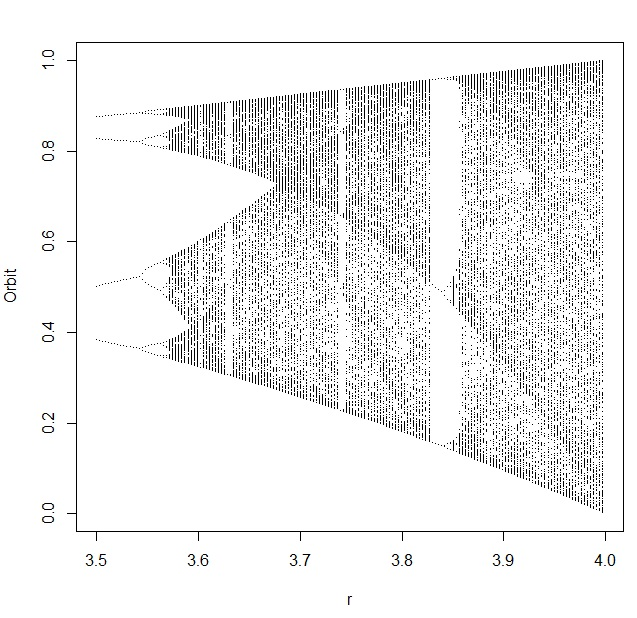If you’ve ever wondered how logistic population growth (the Verhulst model), S curves, the logistic map, bifurcation diagrams, sensitive dependence on initial conditions, “orbits”, deterministic chaos, and Lyapunov exponents are related to one another… this post explains it in just 10 steps, each with some code in R so you can explore it all yourself. I’ve included some code written by other people who have explored this problem (cited below) as portions of my own code.

It all starts with a hypothesized population… and a process where the size of the population changes over time. We want to understand how (and under what conditions) those changes occur, so we choose a model that characterizes population changes: the logistic growth model. It’s been used in biology, ecology, econometrics, marketing, and other areas.

1. The logistic growth model describes how the size of a population (N) changes over time (t), based on some maximum population growth rate (r). There is a limiting factor called the carrying capacity (K) which represents the total population that the environment could support, based on the amount of available resources. dN/dt is the rate of change of the population over time.2. You can simplify the logistic growth model by defining a new variable x to represent the portion of the population that’s alive, compared to the total population that the environment could support (and keep alive). So with x = N/K, you get a new differential equation in terms of x. Now we are looking at the rate of change of the population fraction over time. Once x = N/K = 1, the environment can’t support any more members in the population: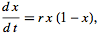3. You can solve this equation by integration! Then, you’ll have an expression that you can use to calculate x (which is still the population fraction) for any time t. This is called the sigmoid or (more commonly), the S Curve. To compute x at any time t, all we need to know is how big the population was when we started looking at it (x0) and the maximum growth rate r:4. The equation for the S Curve is deterministic and continuous. If we want to solve it numerically, we have to discretize it by chopping up that continuous axis that contains time into little tiny pieces of time. That’s what produces the difference equation that we recognize as the logistic map. It’s a map because it “maps” each value of the sequence onto the next value in the sequence. As long as you know one of those values for x (indicated by the subscript n), you’ll be able to figure out the next value of x (indicated by the subscript n+1). The value x[n] is the population fraction of the current generation, and the value x[n+1] is the population fraction for the next generation. This makes the logistic map a Markov chain. If you plot x[n] on the x axis and x[n+1] on the y axis, this expression will produce the familiar upside down parabola: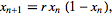5. The logistic map behaves differently depending upon the maximum growth rate (r) that describes your population. This parameter is also called fecundity and represents how rabbit-like your population is reproducing. The higher the r, the more productive, like rabbits (although I’m not sure precisely which r you’d choose if you were studying rabbits). Here is an R function that you can use to generate the last M iterations from a sequence of N total, developed and described at Mage’s Blog:

```logistic.map <- function(r, x, N, M) {
## from http://www.magesblog.com/2012/03/logistic-map-feigenbaum-diagram.html
## r: bifurcation parameter
## x: initial value, something greater than 0 and less than 1
## N: number of iterations (total)
## M: number of iteration points to be returned
z <- 1:N
z <- x
for(i in c(1:(N-1))){
z[i+1] <- r *z[i] * (1 - z[i])
}
## Return the last M iterations
z[c((N-M):N)]
}```

6. The logistic map has many interesting properties, but here are two in particular (the first in Step 6 and the second in Step 7). First, for several values you can choose for r, the chain converges to a single value (or fixed point) when n gets really big. For other values of r, the value of x will eventually bounce between two values instead of converging (a limit cycle of 2). For other values of r, the value of x will eventually bounce between four values instead of converging. Sometimes, x will bounce around a near limitless collection of values (a condition called deterministic chaos). The eventual values (or collection of eventual values, if they bounce between values) is called an orbit. For example, when the growth rate r is 2.6, the logistic map rapidly converges to an orbit of about 0.615:

`plot(logistic.map(2.6,.01,20,20), type="l")`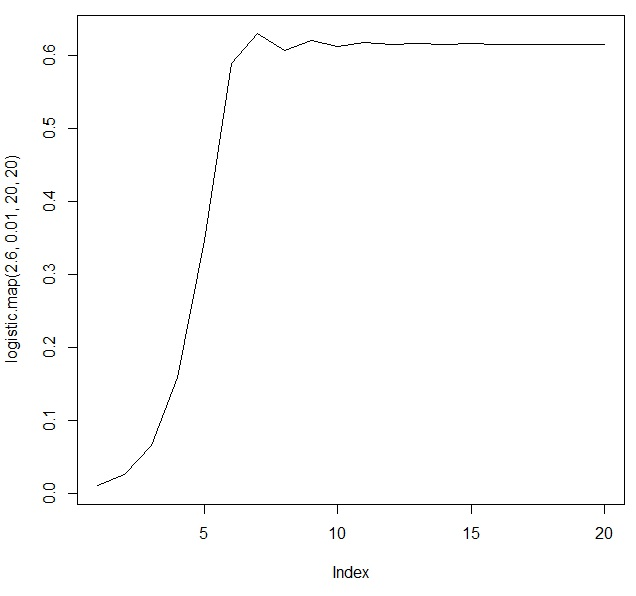7. Sometimes, it can be nice to take a look at how the values bounce around, and where they eventually converge (or not). To do this, we use cobweb diagrams (which are also sometimes called web diagrams). I used a function that I found at http://bayesianbiologist.com to plot the behavior of the orbits for r=2.6, r=3.2, and r=3.9:

```logistic.cobweb <- function(r) {
x<-seq(0,1,length=100)
x_next <- lapply(2:N, function(i) r*x[i]*(1-x[i]))
plot(x[2:N],x_next,type="l",xlim=c(0,1), ylim=c(0,1), main=paste("r=",r),
xlab=expression(x[t]),ylab=expression(x[t+1]), col="red", lwd=2)
abline(0,1)

start=runif(1,0,1)
vert=FALSE
lines(x=c(start,start),y=c(0,r*start*(1-start)) )
for(i in 1:(2*N)) {
if(vert) {
lines(x=c(start,start),y=c(start,r*start*(1-start)) )
vert=FALSE
} else {
lines(x=c(start, r*start*(1-start)), y=c(r*start*(1-start), r*start*(1-start)) )
vert=TRUE
start=r*start*(1-start)
}
}
}

par(mfrow=c(1,3))
logistic.cobweb(2.6)
logistic.cobweb(3.3)
logistic.cobweb(3.9)```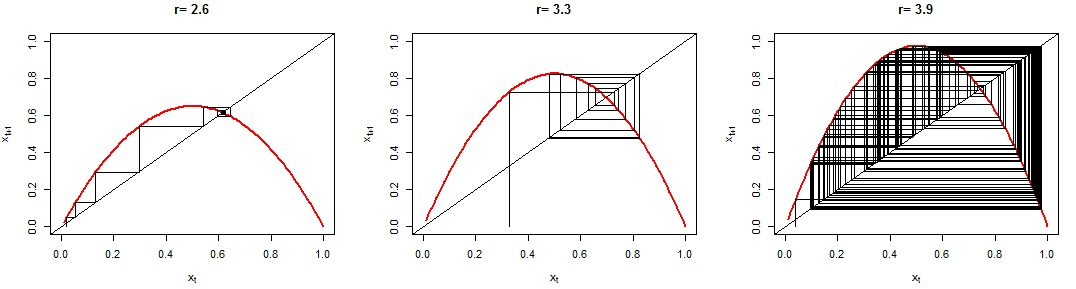8. (Remember to dev.off() before you continue.) Second, for some values of r, the logistic map shows sensitive dependence on initial conditions. For example, let’s see what happens for two different growth rates (r=3 and r=3.9) when we start one iteration with an x[n]  of 0.5 COLORED BLACK, and another one with an x[n] of 0.5001 COLORED RED. It’s a small, small difference that can lead to big, BIG variations in the orbits. In the r=3 case, the chain produced by the logistic map with x[n] of 0.5 (in black) is IDENTICAL to the chain produced by the logistic map with x[n] of 0.5001 (in red). That’s why you can’t see the black… the values are the same! But for the r=3.9 case, the chain produced by the logistic map with x[n] of 0.5 (in black) RAPIDLY DIVERGES from the chain produced by the logistic map with x[n] of 0.5001 (in red). They are very different, despite a very tiny difference in initial conditions! The logistic map for r=3.9 shows a very sensitive dependence on initial conditions

```par(mfrow=c(2,1))
first <- logistic.map(3,.5,120,100)
second <- logistic.map(3,.5001,120,100)
plot(1:length(first),first,type="l",main="r=3 is not sensitive to initial conditions")
lines(1:length(second),second,type="l",col="red")
first <- logistic.map(3.9,.5,120,100)
second <- logistic.map(3.9,.5001,120,100)
plot(1:length(first),first,type="l",main="but r=3.9 is EXTREMELY sensitive")
lines(1:length(second),second,type="l",col="red")

```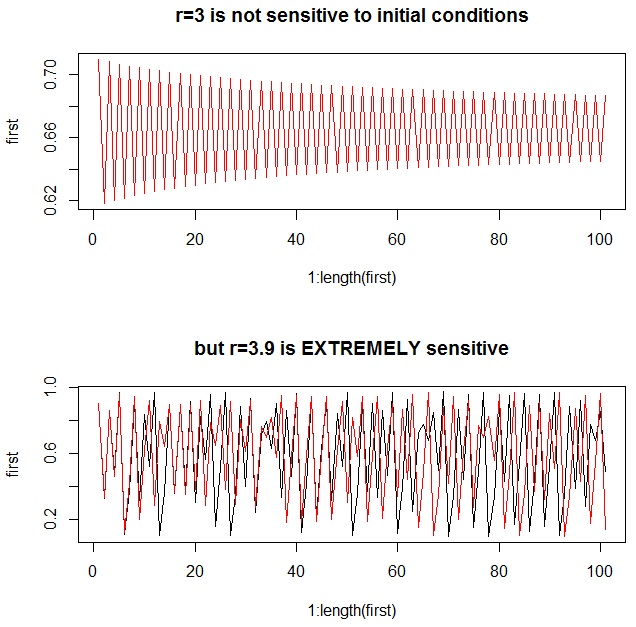9. For any chain, we can determine just how sensitive the logistic map is to initial conditions by looking at the Lyapunov exponent. Very simplistically, if the Lyapunov exponent is negative, the chain will converge to one or more fixed points for that value of r. If the Lyapunov exponent is positive, the chain will demonstrate deterministic chaos for that value of r. If the Lyapunov exponent is zero, there is a bifurcation: a 1-cycle is doubling to a 2-cycle, a 2-cycle is doubling to a 4-cycle, or so forth. The top chart shows an approximation of the Lyapunov exponent based on the first 500 iterations (ideally, you’d use an infinite number, but that would eat up too much computing time), and the bottom chart shows a bifurcation diagramYou’ll notice that the Lyapunov exponents are zero where a bifurcation occurs. To interpret the bifurcation diagram, just remember that each vertical slice through it represents the results of ONE COMPLETELY CONVERGED CHAIN from the logistic map. So it shows the results from many, many, many completely converged chains – and provides an excellent way for us to look at the behavior of MANY different types of populations in just one chart:

```n <- 400
XI <- lya <- 0
x <- seq(0,4,0.01)
for (i in 1:n) {
xi <- logistic.map(x[i],.01,500,500)
XI <- rbind(XI,xi)
}
for (i in 1:length(x)) {
lya[i] <- sum(log(abs(x[i]-(2*x[i]*XI[i,]))))/length(x)
}
plot(x,lya,ylim=c(-4,1),xlim=c(0,4),type="l",main="Lyapunov Exponents for Logistic Map")
abline(h=0, lwd=2, col="red")
# next 3 lines from http://www.magesblog.com/2012/03/logistic-map-feigenbaum-diagram.html:
my.r <- seq(0, 4, by=0.003)
Orbit <- sapply(my.r, logistic.map, x=0.1, N=1000, M=300)
r <- sort(rep(my.r, 301))

par(mfrow=c(2,1))
plot(x,lya,ylim=c(-5,1),xlim=c(0,4),type="l",main="Lyapunov Exponents for Logistic Map")
abline(h=0, col="red", lwd=2)
abline(v=3, col="blue", lwd=2)
plot(r, Orbit, pch=".", cex=0.5, main="Bifurcation Diagram for r=0 to r=4 Logistic Maps")
abline(v=3, col="blue", lwd=2)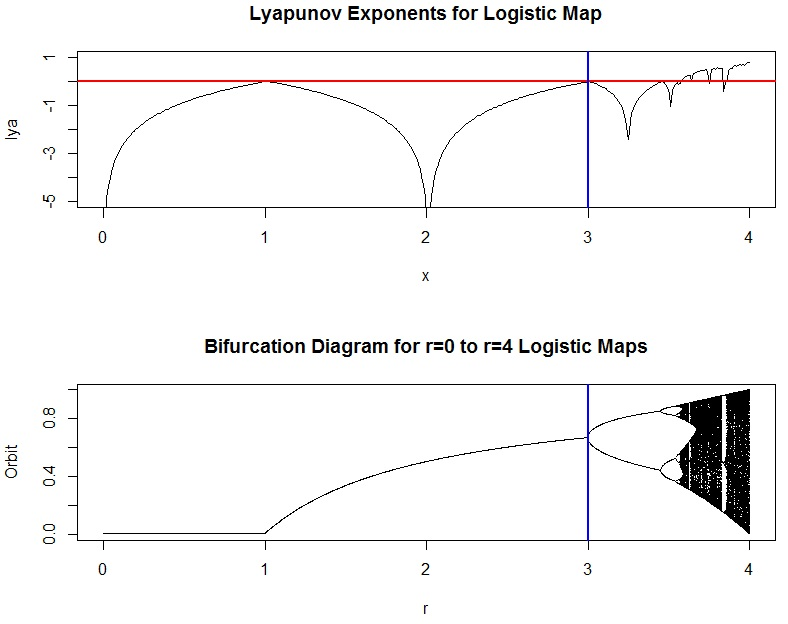```

10. Notice that in the bifurcation diagram, we can easily see that when r is between 0 and 1, the population converges to extinction. This makes sense, because the growth rate is smaller than what’s required to sustain the size of the population. You might like to zoom in, though, and see what the orbits look like for some smaller portions of the diagram. Here’s how you can do it (but be sure to refresh your graphics window with dev.off() before you try it). Try changing the plot character (pch) too, or maybe the size of the characters with cex=0.2 or cex=0.5 in the last line:

```# adapted from http://www.magesblog.com/2012/03/logistic-map-feigenbaum-diagram.html:
my.r <- seq(3.5, 4, by=0.003)
Orbit <- sapply(my.r, logistic.map, x=0.1, N=1000, M=300)
multiplier <- length(Orbit)/length(my.r)
r <- sort(rep(my.r, multiplier))
plot(r, Orbit, pch=".")```That’s it!

Find out more information on these other web pages, which are listed in order of difficulty level:

## What is Innovation? Towards a Universal DefinitionWhat is innovation? It’s become such an overused management buzzword over the past couple decades that, when I told my very esteemed executive woman friend that I was planning to write a book on innovation, she groaned. “Don’t do that,” she said. “Everybody does that.”

Just today, Fast Company published an article asserting that we really need a commonly accepted definition for innovation to “weed out the truly innovative from the rest”. Its author, Stephen Uban, goes on to explain that the solution to this dilemma is the (apparently new) Innovation Standard that has been developed by the Product Development and Management Association (PDMA).

Is innovation a new product line? Does it represent an improved process for efficiency? Is it a great idea?